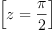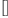login  home  contents  what's new  discussion  bug reports help  links  subscribe  changes  refresh  edit

 Submitted by : (unknown) at: 2007-11-17T21:51:45-08:00 (15 years ago) Name : Axiom Version : default friCAS-20090114 Axiom-20050901 OpenAxiom-20091012 OpenAxiom-20110220 OpenAxiom-Release-141 Category : Axiom Aldor Interface Axiom Compiler Axiom Library Axiom Interpreter Axiom Documentation Axiom User Interface building Axiom from source lisp system MathAction Doyen CD Reduce Axiom on Windows Axiom on Linux Severity : critical serious normal minor wishlist Status : open closed rejected not reproducible fix proposed fixed somewhere duplicate need more info Optional subject :   Optional comment :

Branch cuts differ?

fricas
(1) -> )clear all
All user variables and function definitions have been cleared.
solve(cot(z)=0, z)(1)
Type: List(Equation(Expression(Integer)))
fricas
solve(cos(z)/sin(z)=0, z)(2)
Type: List(Equation(Expression(Integer)))

(27) -> solve(cos(z)/sin(z)=0, z)   (27)  []
Type: List Equation Expression Integer
(28) -> solve(cot(z)=0, z)             %pi
(28)  [z= ---]
2
Type: List Equation Expression Integer


Mathaction display problem? (Above verbatim text is not shown)

fricas
)set output algebra on

fricas
)set output tex off

fricas
solve(cos(z)/sin(z)=0, z)
(3)  []
Type: List(Equation(Expression(Integer)))
fricas
solve(cot(z)=0, z)
%pi
(4)  [z = ---]
2
Type: List(Equation(Expression(Integer)))

Mathaction display problem? --Bill Page, Thu, 17 Feb 2005 15:30:42 -0600 reply
Yes, ever since I upgraded to a new version of the Python Image Library (last week), MathAction seems to occassionally go into a mode where it cannot render LaTeX images properly. I do not yet know the cause however with a little tweaking (like restarting the web server and deleteing the improperly generated images), I can manage to get it to work for a while. (I have made such corrections to this page.)

I am investigating the problem now. Please be patient. Thanks.

property change
Tue, 08 Mar 2005 05:01:25 -0600 reply

Category: Axiom Mathematics => Axiom Library

 Subject:   Be Bold !! ( 14 subscribers )